# Reason + natural numbers - math problems

#### Number of problems found: 238

• Unknown numberI think the number: Its half is 16 bigger than his quarter. I think the number N . ..
• Sum and roundingI know two numbers whose sum is 20. When they each round and then added together I get the 30. What are this numbers?
• Foot in busIt was 102 people on the bus. 28 girls had two dogs. A 11 girls had one dog. At the next stop seceded 5 dogs (even with their owners). They got two boys together with three dogs. The bus drove one driver. How many foot were in bus?
• Digits of age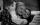The product of the digits of Andrew age as 6 years ago and not equal to 0. Andrew's age is also the youngest possible age with these two conditions. After how many years will the product of the digits of Andrew age again the same as today?In six baskets, the seller has fruit. In individual baskets, there are only apples or just pears with the following number of fruits: 5,6,12,14,23 and 29. "If I sell this basket," the salesman thinks, "then I will have just as many apples as a pear." Whic
• Cents no more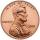Janko bought pencils for 35 cents each. Neither he nor the salesperson had small coins, just a whole € 1 coin. At least how many pencils had to buy to pay for the whole euros?
• Landlord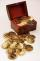Landlord had 49 ducats more than Jurošík. How many ducats Jurošík steal landlord if the Jurošík now 5 ducats more?
• Florist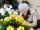Florist has 84 red and 48 white roses. How many same bouquets can he make from them if he must use all roses?
• Number train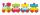The numbers 1,2,3,4,5,6,7,8 and 9 traveled by train. The train had three cars and each was carrying just three numbers. No. 1 rode in the first carriage, and in the last carriage was all odd numbers. The conductor calculated sum of the numbers in the firs
• Sheep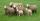Shepherd tending the sheep. Tourists asked him how much they have. The shepherd said, "there are fewer than 500. If I them lined up in 4-row 3 remain. If in 5-row 4 remain. If in 6-row 5 remain. But I can form 7-row." How many sheep have herdsman?
• RectangleThe perimeter of the rectangle is 22 cm and content area 30 cm2. Determine its dimensions, if the length of the sides of the rectangle in centimeters is expressed by integers.
• Trees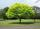Loggers wanted to seed more than 700 and less than 800 trees. If they seed in rows of 37, left them 8 trees. If they seed in rows of 43, left the 11 trees. How many trees must seed ?
• Basements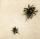In the first basement is more flies than the spiders, the second vice versa. Each basement had spiders and flies together 100 feet. Determine how many could be flies and spiders in the first and second basement. PS. We only need, when you write how many s
• Exhibition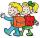The teacher paid 280 Kč for 4.A students for admission to the exhibition. How many students were at the exhibition?
• Unknown numberSamuel wrote unknown number. Then he had add 200000 to the number and the result multiply by three. When it calculated he was surprised, because the result would have received anyway, if write digit to the end of original number. Find unknown number.
• Digits A, B, CFor the various digits A, B, C is true: the square root of the BC is equal to the A and sum B+C is equal to A. Calculate A + 2B + 3C. (BC is a two-digit number, not a product).
• Four-digit numberFind also a four-digit number, which quadrupled written backwards is the same number.
• Unknown number 5I think of an unknown number. If we enlarge it five times, then subtract 3, and the result decreases by 75%, we get one greater than the number. What number am I thinking of?
• Florist's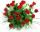The florist got 72 white and 90 red roses. How many bouquets can bind from all these roses when each bouquets should have the same number of white and red roses?
• Unknown number 10Number first increased by 30%, then by 1/5. What percentage we've increased the original number?

Do you have an exciting math question or word problem that you can't solve? Ask a question or post a math problem, and we can try to solve it.

We will send a solution to your e-mail address. Solved examples are also published here. Please enter the e-mail correctly and check whether you don't have a full mailbox.

Reason - math word problems. Natural numbers - math word problems.